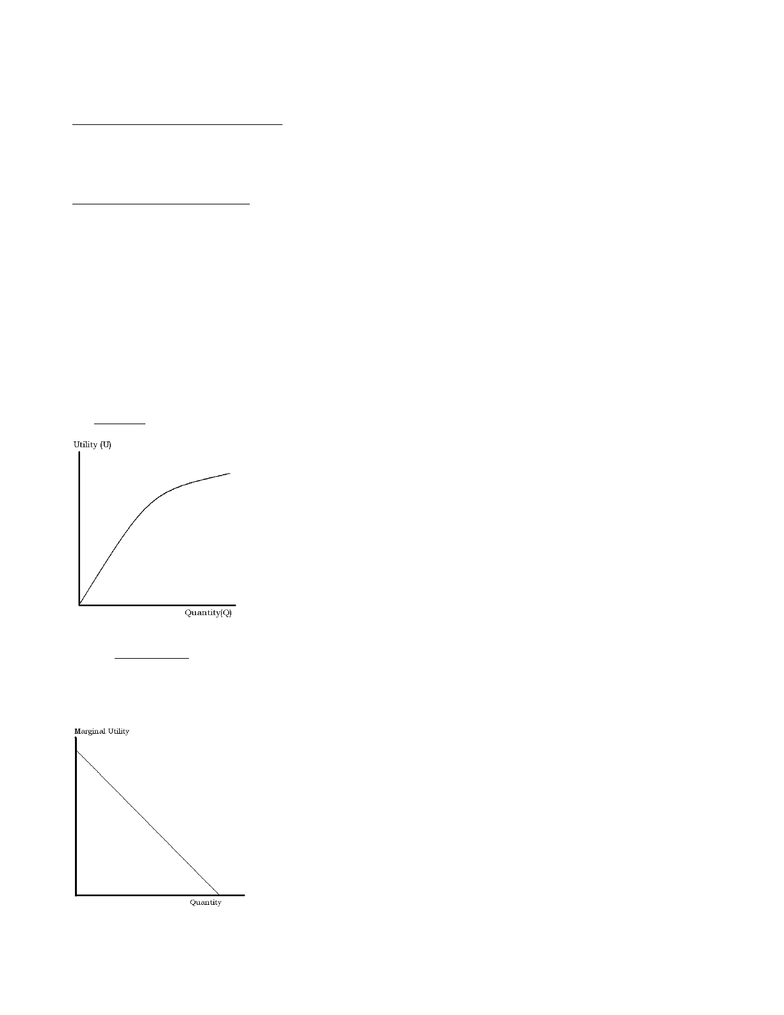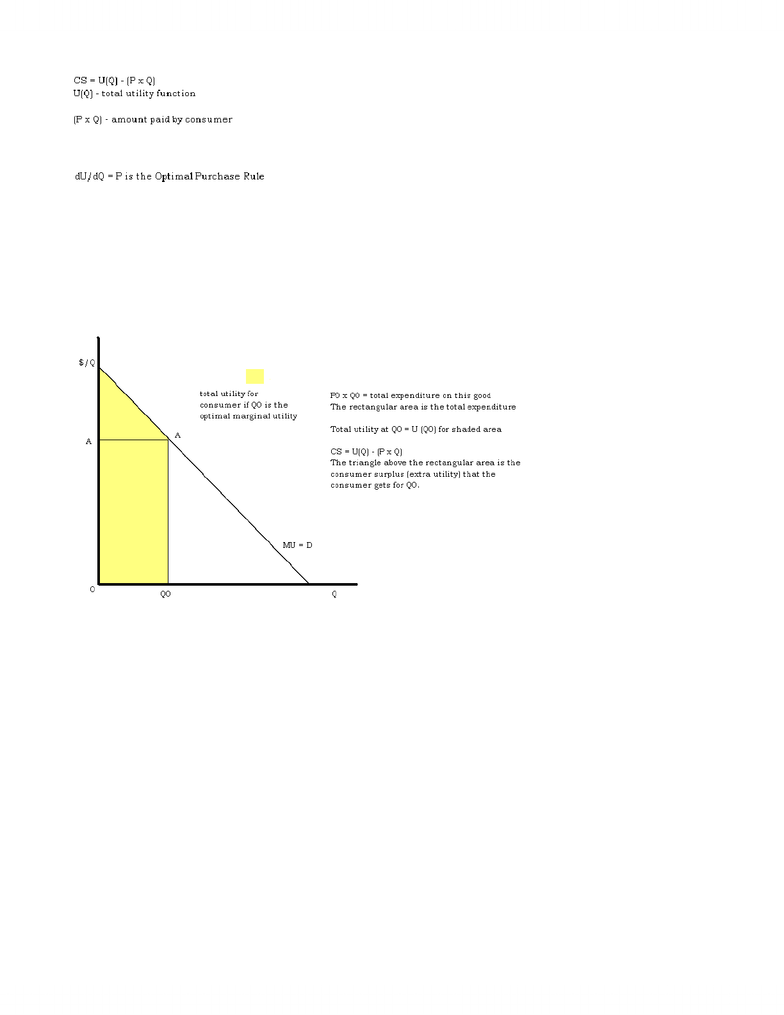# Lecture notes week 3

40 views6 pagesWeek #3 – Demand and Utility
Review from last week:
Demand and Supply in a Competitive Market
Behaviour of buyers and behaviour of sellers are both affected by the price of the good. Movements in price will bring these planned
behaviours together in equilibrium. Graphically, changes in price cause movements along demand and supply curves.
Changes in any underlying factor cause shifts in these demand or supply curve. For demand curves, these underlying factors include price
of a substitute, price of a complement, consumer incomes, population and tastes. For supply curves, the underlying factors are the price
of labour, the price of capital, the price of other inputs, and technology.
Explaining/Exploring Demand Curves
Why are demand curves negatively sloped?
How do we interpret a point on the demand curve?
How do we interpret an area under the demand curve?
How do demand curves relate to behaviour of consumers and well-being of consumers?
The following points are some of the answers that economists give to the question of why people consume.
x Demand curves reflect theutility” (or well-being, or satisfaction, or happiness) of consumers – measured in dollars.
x Demand curves can be used to measure the willingness-to-pay for a good by consumers.
x Individuals who consume are making decisions designed to maximize their own well-being (utility), with the income they have
available.
x Utility is usually not measured in dollars, it is measured in utils
An example for these points is as follows:
Imagine Fred has a utility function for hamburgers per month where Q = # of hamburgers.
We assume (a bit unusual, but convenient) that U is measured in dollars (the value of well-being to Fred).
Fred’s utility function § U = 20Q - Q2
U is total utility
Draw and label the total utility function. Notice its shape.
Perhaps you can see that dU/dQ = 20 - 2Q
dU/dQ is marginal utility
Marginal utility always refers to derivatives
Marginal utility is positive, but as Q increases, this gets smaller (it diminishes – d2U/dQ2 < 0 - this is called [the assumption of]
diminishing marginal utility)
Draw and label the marginal utility function. Notice its shape. Marginal utility is downward sloping
Fred wants to maximize his net gain from consuming hamburgers. He gains utility from consuming hamburgers, but (/) Fred has to pay
for the hamburgers. The net gain is the difference between these is called Consumer Surplus (CS)
www.notesolution.com
Unlock document

This preview shows pages 1-2 of the document.
Unlock all 6 pages and 3 million more documents.Fred seeks to maximize CS.
Maximize by: dCS/dQ = dU/dQ – P = 0
Therefore, when dU/dQ = P, CS is maximized. When marginal utility = price, CS is maximized.
Rational consumers follow the Optimal Purchase Rule, and the demand curve is the result. The demand curve and MU curve are the same.
In words, consumers choose to consume hamburgers up until the point where the (diminishing) marginal utility of consuming those
hamburgers is equal to the price of hamburgers.
If we take all possible points for Fred where dU/dQ = P, this will give us Fred’s monthly demand curve for hamburgers.
For Fred, this gives us P = dU/dQ = 20 – 2Q
Then if P = \$2, 2Q = 18 or Q = 9
If P = \$4, 2Q = 16 or Q = 8
If P = \$6, 2Q = 14 or Q = 7
If P = \$20, 2Q = 0 or Q = 0
www.notesolution.com
Unlock document

This preview shows pages 1-2 of the document.
Unlock all 6 pages and 3 million more documents.

## Document Summary

Behaviour of buyers and behaviour of sellers are both affected by the price of the good. Movements in price will bring these planned behaviours together in equilibrium. Graphically, changes in price cause movements along demand and supply curves. Changes in any underlying factor cause shifts in these demand or supply curve. For demand curves, these underlying factors include price of a substitute, price of a complement, consumer incomes, population and tastes. For supply curves, the underlying factors are the price of labour, the price of capital, the price of other inputs, and technology. The following points are some of the answers that economists give to the question of why people consume. N demand curves reflect the utility (or well-being, or satisfaction, or happiness) of consumers measured in dollars. N demand curves can be used to measure the willingness-to-pay for a good by consumers.

## Get access

\$10 USD/m
Billed \$120 USD annuallyHomework Help
Study Guides
Textbook Solutions
Class Notes
Textbook Notes
Booster Class